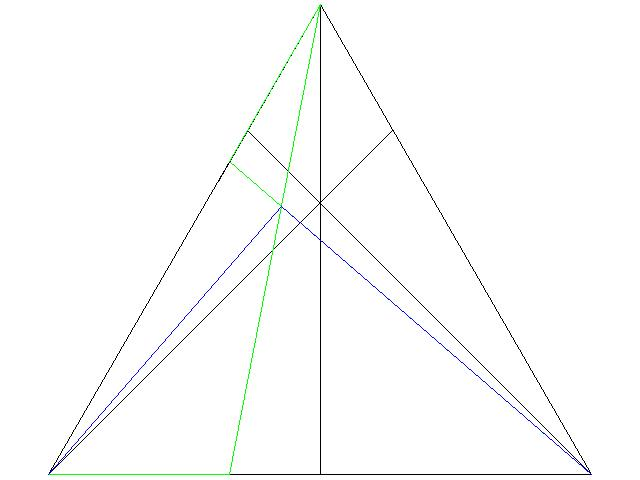4) ABC is an equilateral triangle. D is a point on segment CB such that DB is half as long as DC. E is a point on segment AB such that EA is half as long as EB. F is the point of intersection of the segments AD and CE. Prove: angle CFB is 90 degrees. [this is a good example of a proof where the coordinate geometry proof is straightforward but the classical geometry proof is quite difficult and very instructive to follow. I say to follow, because most people would not be able to do it on their own, but it is quite instructive to read and study the proof]. This is a problem sent to me by my email friend C. Blanco. He is always looking for challenging problems. He believes it is better to work on possible problems as compared to something nearly impossible such as a Prime number series. I have to agree with him. There is much new knowledge to be learned solving attainable solutions. You learn from them and you pick up new math tools for your toolkit. Below is my attempt at a solution. It is not a complete proof but may create ideas for a different approach to the problem.We know that since it is an equilateral triangle the 3 angles are each 60 degrees. And we know that we can form a 90-45-45 degree triangle inside of the bottom 60 degree angles. (I marked it as 30 deg in the picture but latter realized my mistake.) Now the fun part. We rotate this 90 degree angle around point A. As we rotate we are subtracting length from side B while adding length to side C. But we must make sure to maintain the 90 degree angle. It is sort of complex but think of a vector table like the ones used in physics class. As you pull one side the other moves. But in this case we are changing the lengths of those vectors instead of moving them. We are basically working on the fact that a 45—45 right triangle has known sides so it is useful in are attempt to solve the triangle. We sort of “morph” the 45—45 right triangle so that the length of the sides change, but the 90 degree angle stays the same. It is sort of like using the 45—45 right triangle as a relative coordinate system. Note that this problem could be easily solved using trigonometry to find the sides. In fact many attempts have lead myself to what the definition of the sine and cosine are. But I want to use nothing but classic geometry. I will only use the Pythagorean theorem. I doubt my solution is what the designer of the problem had in mind. They probably used logic. However after a long while of looking at this problem, I do not see it. But that is what makes math so fun.NOTE: This is an ongoing project. I will update the site with better drawings once I am sure the solution is answering the problem. I already see one mistake that in should be 1/2 (c-a). But the problem will prove to work or just be an interesting idea. I know that better drawings of the steps are needed. Stay posted to Constructor's Corner for updates.

May the Creative Force be with You